# 模糊数学基础

【114python之家】模糊数学基础文章目录模糊数学基础1.前言2.区分随机性和模糊性3.模糊数学的基本概念1.模糊集和隶属函数2.模糊集的表示3.确定隶属函数的方法4.与传统集合论的区分4.模糊数

# 模糊数学基础

### 文章目录

• 模糊数学基础
• 1.前言
• 2.区分随机性和模糊性
• 3.模糊数学的基本概念
• 1.模糊集和隶属函数
• 2.模糊集的表示
• 3.确定隶属函数的方法
• 4.与传统集合论的区分
• 4.模糊数学的基本运算
• 1.模糊集的运算
• 2.模糊关系与运算
• 1.关系与模糊关系
• 2.模糊关系矩阵的运算
• 3.python程序求解法

## 1.前言

1965年美国著名控制论专家发表了FuzzySets从而开创了模糊数学的基本概念用“隶属度”和“隶属函数”来描述差异的中间过渡，处理和刻画模糊现象.

1. 确定性数学模型
2. 随机性数学模型
3. 模糊性数学模型

## 3.模糊数学的基本概念

​M:U->[0,1],

​u->M(u),

### 2.模糊集的表示

​当论域U为有限集时，记U={u1,u2,···,un},则U上的模糊集M有下列三种常见表现形式.

1. 序偶表示法

M = ( u 1 , M ( u 1 ) ) , ( u 2 , M ( u 2 ) ) , ⋅ ⋅ ⋅ , ( u n , M ( u n ) ) . M={(u1,M(u1)),(u2,M(u2)),···,(un,M(un))}. M=(u1,M(u1)),(u2,M(u2)),,(un,M(un)).

2. 向量表示法

M = ( M ( u 1 ) , M ( u 2 ) , ⋅ ⋅ ⋅ M ( u n ) ) . M=(M(u1),M(u2),···M(un)). M=(M(u1),M(u2),M(un)).

3. 扎德表示法

M = ∑ i = 1 n M ( u i ) u i = M ( u 1 ) u 1 + M ( u 2 ) u 2 + M ( u 3 ) u 3 + M ( u n ) u n M=sum_{i=1}^{n}frac{M(u_i)}{u_i}=frac{M(u_1)}{u_1}+frac{M(u_2)}{u_2}+frac{M(u_3)}{u_3}+frac{M(u_n)}{u_n} M=i=1nuiM(ui)=u1M(u1)+u2M(u2)+u3M(u3)+unM(un)

注：" ∑ sum ∑“和”+"不是求和的意思，只是表示集合元素的记号. M ( u i ) u i frac{M(u_i)}{u_i} uiM(ui)不是分数，它表示点 u i u_i ui对模糊集M的隶属度是 M ( u i ) M(u_i) M(ui).

当论域U为无限集时，U上的模糊集M可表示为: M = ∫ u ∈ M M ( u ) u M=int_{uin{M}}{frac{M(u)}{u}} M=uMuM(u)

注：" ∫ int ∫"也不是代表积分的意思

### 3.确定隶属函数的方法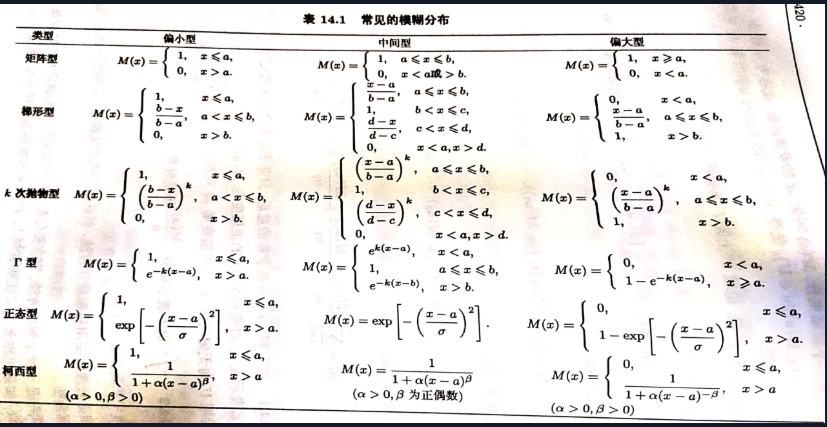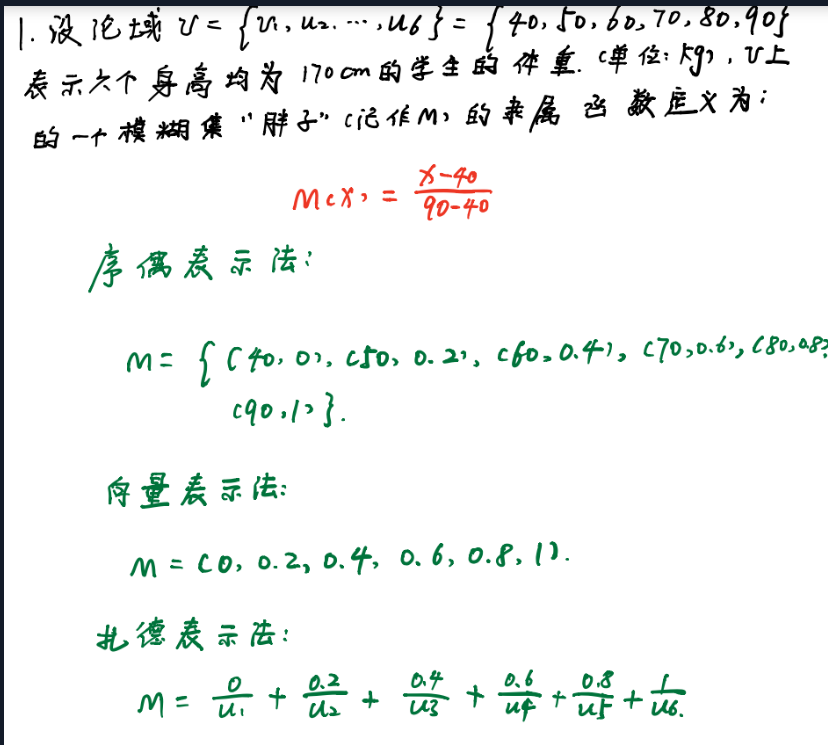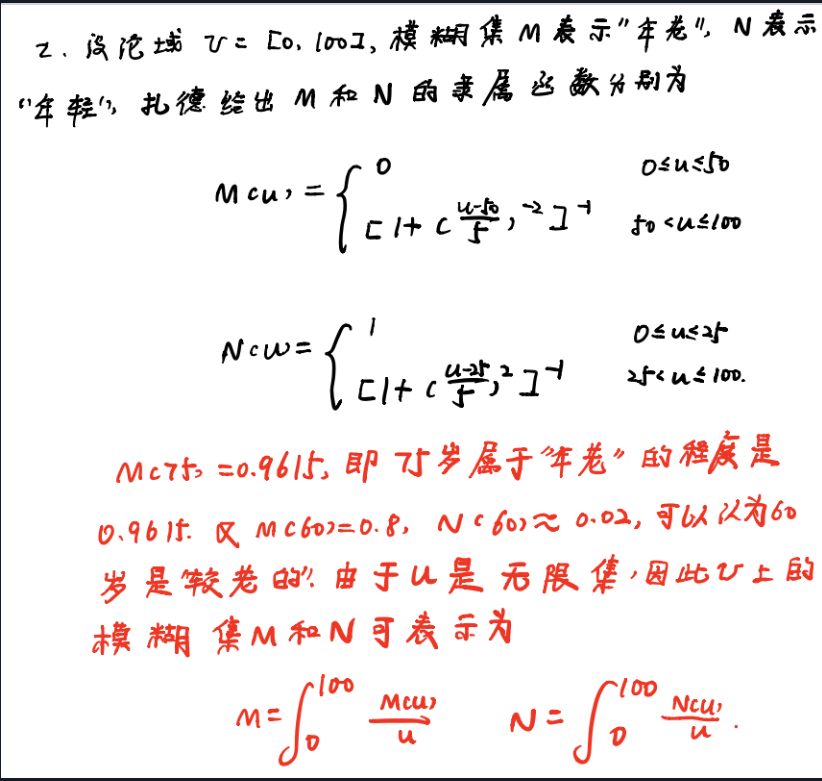## 4.模糊数学的基本运算

### 1.模糊集的运算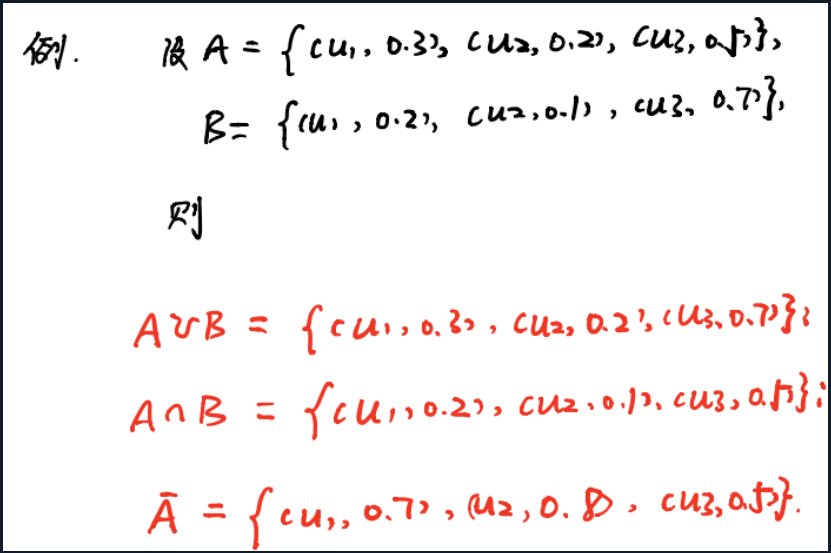### 2.模糊关系与运算

#### 1.关系与模糊关系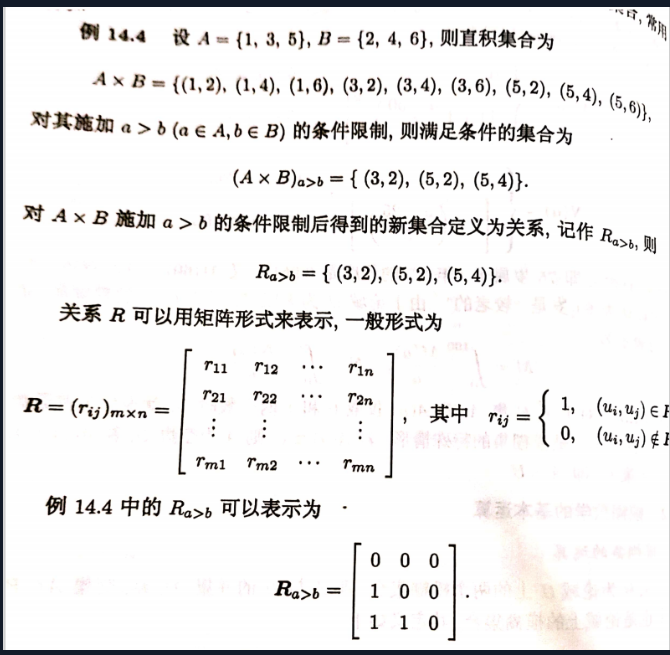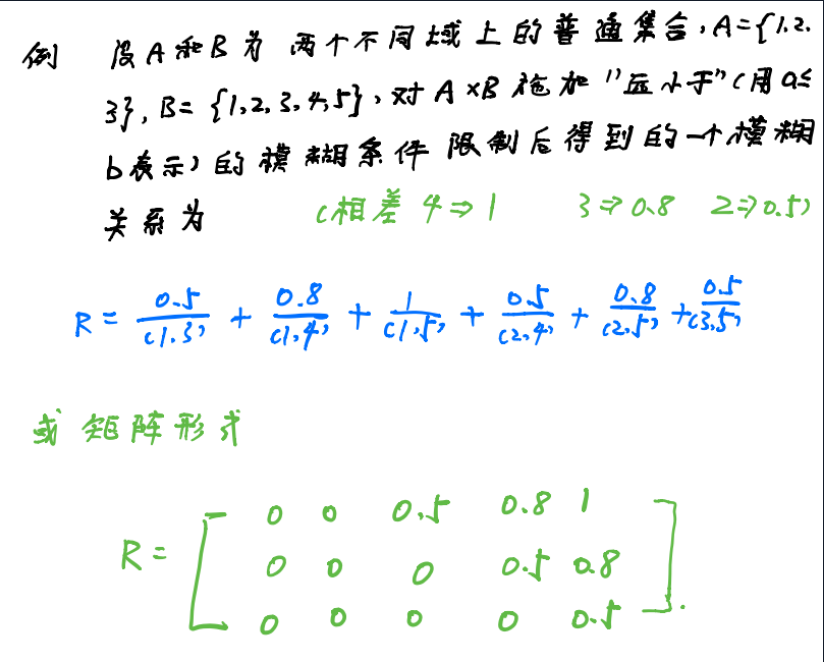#### 2.模糊关系矩阵的运算

R = ( r i j ) m × n , S = ( s i j ) m × n R=(r_{ij})_{m imes{n}},S=(s_{ij})_{m imes{n}} R=(rij)m×n,S=(sij)m×n为同一论域U上的两个模糊关系矩阵，i=1,2,···,m

j=1,2,···,n,则其并、交、补运算分别定义为(因markdown实在不好打出故采用手写)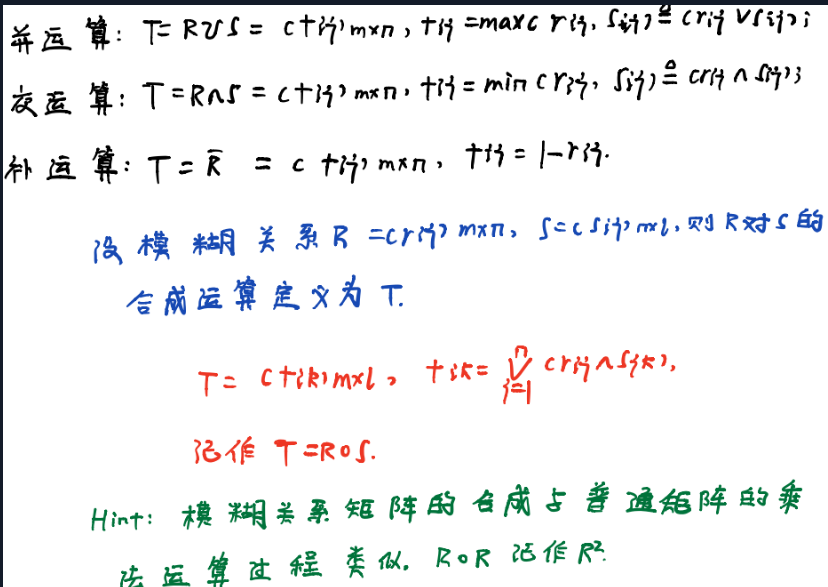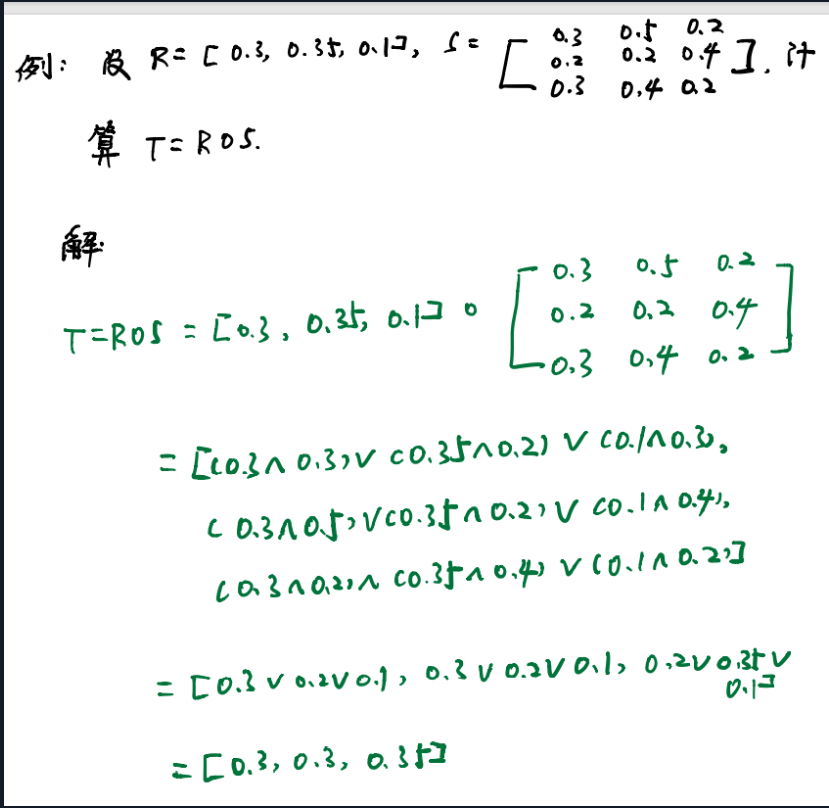#### 3.python程序求解法

importnumpyasnp
a=np.array([0.3,0.35,0.1]);aa=np.tile(a,(len(a),1))#或者说平铺第一个参数为axis=0复制几倍第二个参数为axis=1轴复制几倍使得维度与b匹配
b=np.array([[0.3,0.5,0.2],[0.2,0.2,0.4],[0.3,0.4,0.2]])
#模拟模糊关系矩阵合成运算
c=np.minimun(aa.T,b)#两个矩阵的元素对应求最小值
T=c.max(axis=0)#矩阵逐列求最大值成行向量
print("T=",T)## 评论列表

• 这篇文章还没有收到评论，赶紧来抢沙发吧~